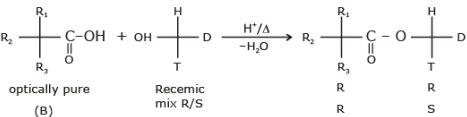Courses

# Alcohols MCQ - 1 (Advanced)

## 25 Questions MCQ Test Chemistry for JEE Advanced | Alcohols MCQ - 1 (Advanced)

Description
This mock test of Alcohols MCQ - 1 (Advanced) for JEE helps you for every JEE entrance exam. This contains 25 Multiple Choice Questions for JEE Alcohols MCQ - 1 (Advanced) (mcq) to study with solutions a complete question bank. The solved questions answers in this Alcohols MCQ - 1 (Advanced) quiz give you a good mix of easy questions and tough questions. JEE students definitely take this Alcohols MCQ - 1 (Advanced) exercise for a better result in the exam. You can find other Alcohols MCQ - 1 (Advanced) extra questions, long questions & short questions for JEE on EduRev as well by searching above.
QUESTION: 1

### Which of the following is the product from ethanol addition to dihydropyran (shown on the left below) ?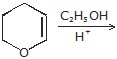Solution: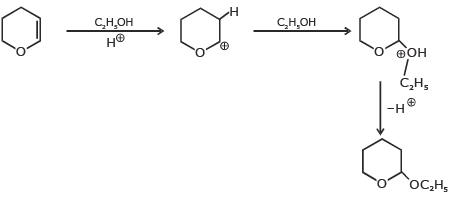QUESTION: 2

### What product (s) are expected from the following reaction ?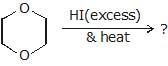Solution: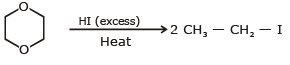QUESTION: 3

### Which of the following reagents would be best for oxidizing a 1º-alcohol to an aldehyde ?

Solution: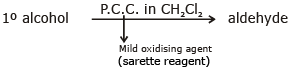QUESTION: 4

A water soluble C6H14O2 compound is oxidized by lead tetraacetate (or periodic acid) to a single C3H6O carbonyl compound. Which of the following would satisfy this fact ?

Solution: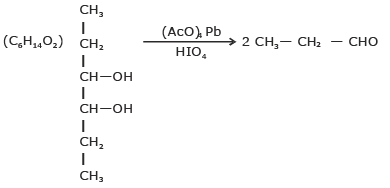QUESTION: 5

A racemic mixture of (±) 2-phenyl propanoic acid on esterificaton with (+) 2-butanol gives two esters. Mention the sterochemistry of two esters formed -

Solution: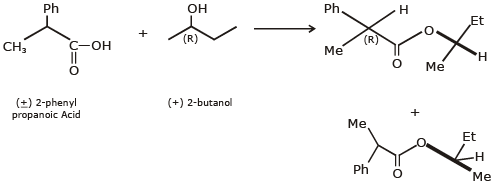*Multiple options can be correct
QUESTION: 6

Compound which gives alcohol on reduction is/are (When reacts with LiAH4)

Solution: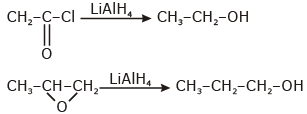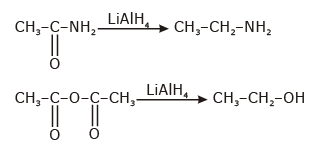*Multiple options can be correct
QUESTION: 7

Which of following compound undergo acidic hydrolysis.

Solution: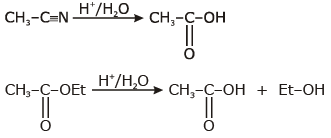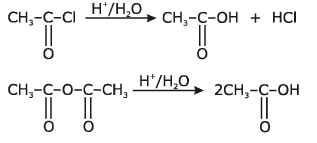*Multiple options can be correct
QUESTION: 8

Which of the following alcohol can not be oxidized by KMnO4 :

Solution: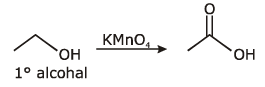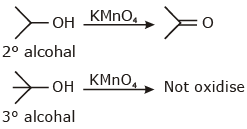*Multiple options can be correct
QUESTION: 9

Which of the following reaction esterification ?

Solution: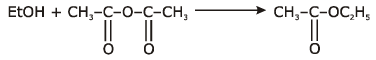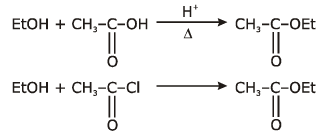*Multiple options can be correct
QUESTION: 10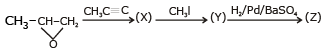Identify X, Y, Z :

Solution: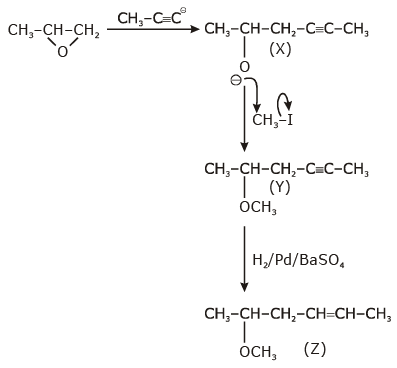*Multiple options can be correct
QUESTION: 11

Which is/are corect statement ?

Solution: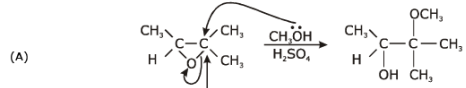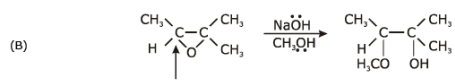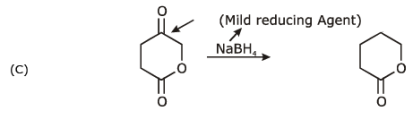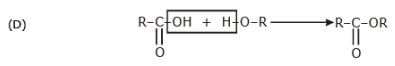*Multiple options can be correct
QUESTION: 12

3-methyl-3-hexanol can be prepared by -

Solution: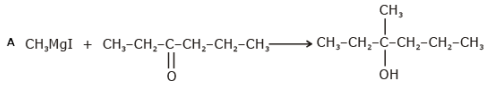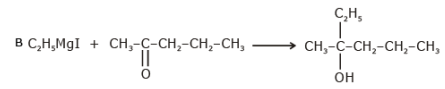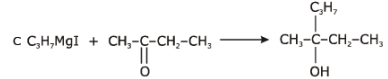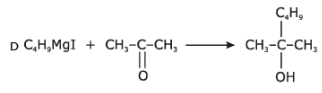*Multiple options can be correct
QUESTION: 13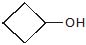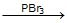(X)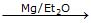(Y)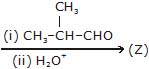Solution: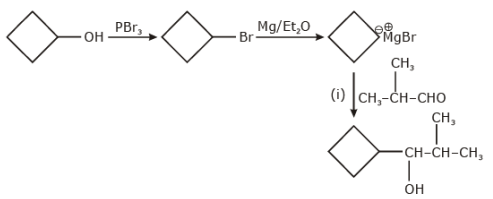*Multiple options can be correct
QUESTION: 14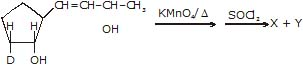Identify the product X and Y.

Solution: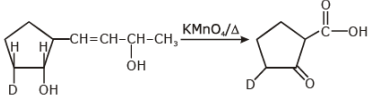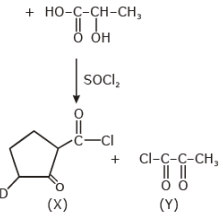*Multiple options can be correct
QUESTION: 15

Aldehydes and ketones react with oine molecule of analcohol to form compounds called hemiacetals, in which there is one hydroyl group and one either-like group. Reaction of a hemiacetal with a second molecule of alcohol gives an acetal and a molecule of water. We study this reaction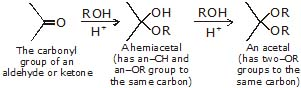Draw structural formulas for the hemicetal and acetal formed from these reagents. The stoichiometry of each reaction is given in the problem.

(A)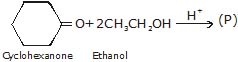(B)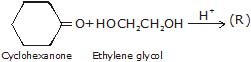(C)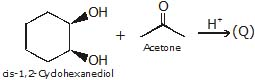Q.

Product P is -

Solution: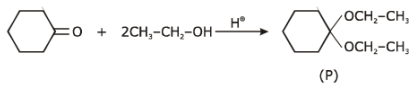*Multiple options can be correct
QUESTION: 16

Aldehydes and ketones react with oine molecule of analcohol to form compounds called hemiacetals, in which there is one hydroyl group and one either-like group. Reaction of a hemiacetal with a second molecule of alcohol gives an acetal and a molecule of water. We study this reactionDraw structural formulas for the hemicetal and acetal formed from these reagents. The stoichiometry of each reaction is given in the problem.

(A)(B)(C)Q.

Product Q is -

Solution: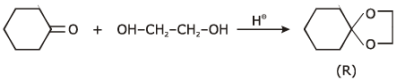*Multiple options can be correct
QUESTION: 17

Aldehydes and ketones react with oine molecule of analcohol to form compounds called hemiacetals, in which there is one hydroyl group and one either-like group. Reaction of a hemiacetal with a second molecule of alcohol gives an acetal and a molecule of water. We study this reactionDraw structural formulas for the hemicetal and acetal formed from these reagents. The stoichiometry of each reaction is given in the problem.

(A)(B)(C)Q.

Product R is -

Solution:

β- hydroxy carbonyl and cis 1-2, diol can be cleave by HIO4.

*Multiple options can be correct
QUESTION: 18

Which can be cleaved by HIO4 ?

Solution:
QUESTION: 19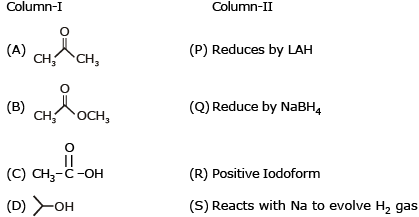Solution:

A → P,Q,R; B → P,C; C → P, S D → R, S

QUESTION: 20

Match the following X, yZ (C5H12O) are isomeric alcohols on oxidation `X' gives a given, Y gives acid and Z is not oxidised X gives positive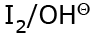test. The activity order with HBr is Z > X > Y.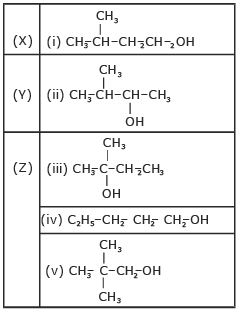Solution:

X ⇒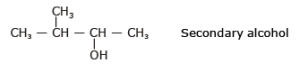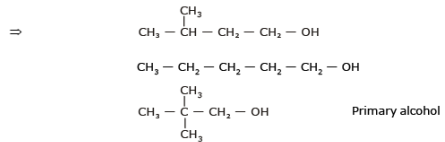Z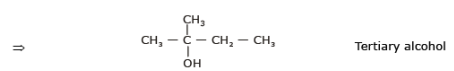A–ii; B–i, iv, v; C – iii

QUESTION: 21

1-propanol & 2-propanol can be best distinguished by -

Solution: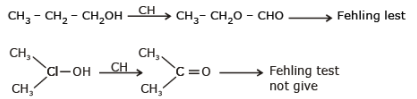QUESTION: 22

Identify the correct order of boiling point of the following compounds :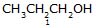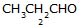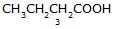[JEE 2002]

Solution:

(1) molecular wright µ boil point
(2)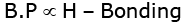QUESTION: 23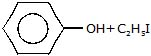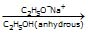Solution: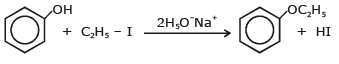QUESTION: 24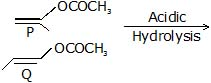Product formed by P & Q can be differentiated by:

Solution: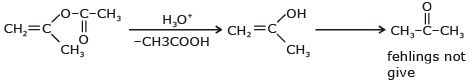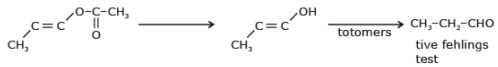QUESTION: 25

Reactn of entainomerically pure acid with 1 chiral carbon and racemic alcohol with 1 chiral carbon gives an ester which is -

Solution: